Examples

Chapter 1 Class 7 Integers
Serial order wise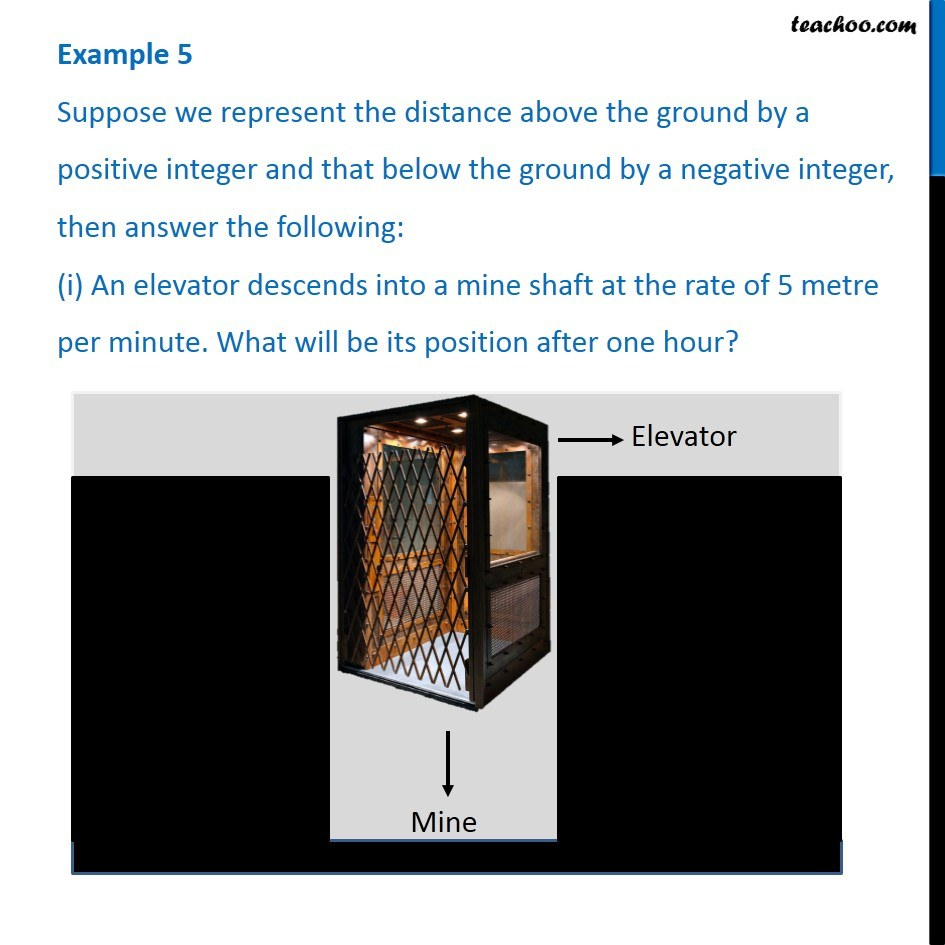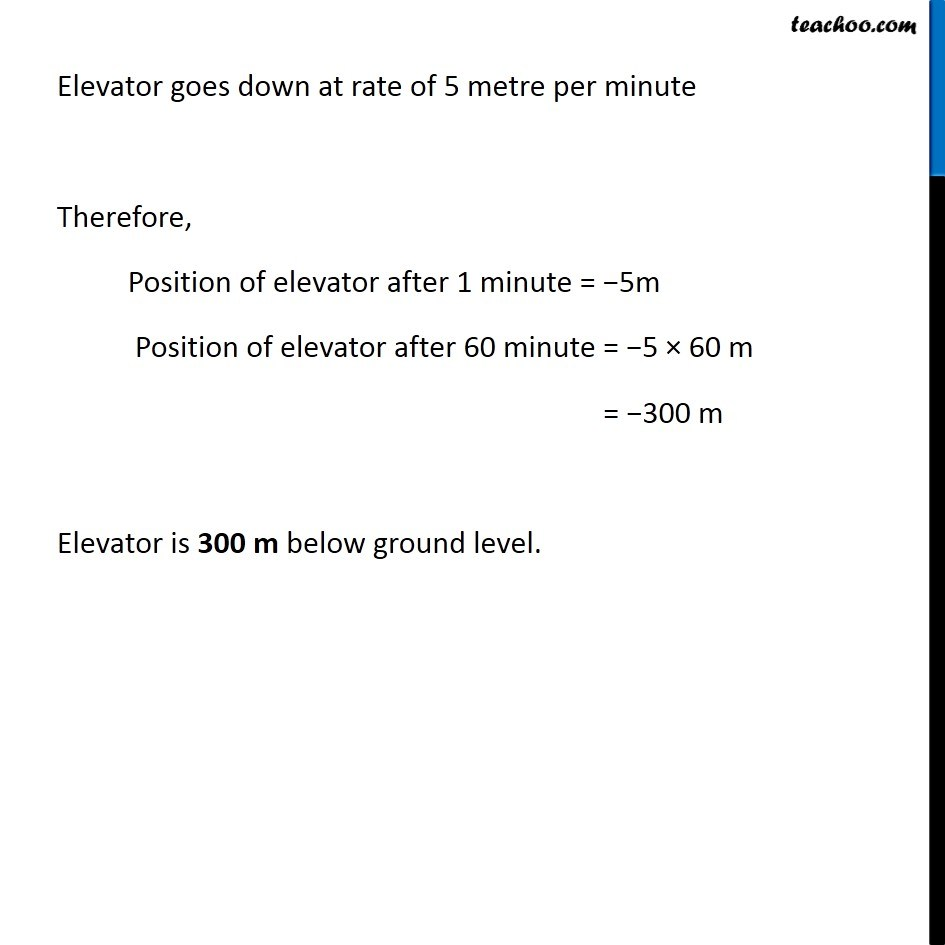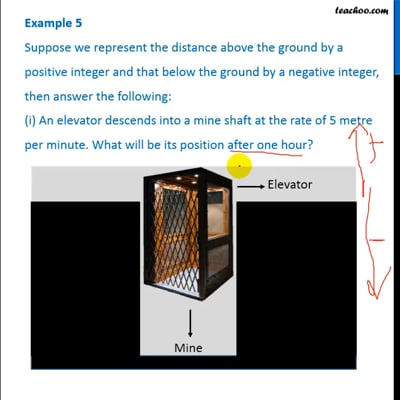This video is only available for Teachoo black users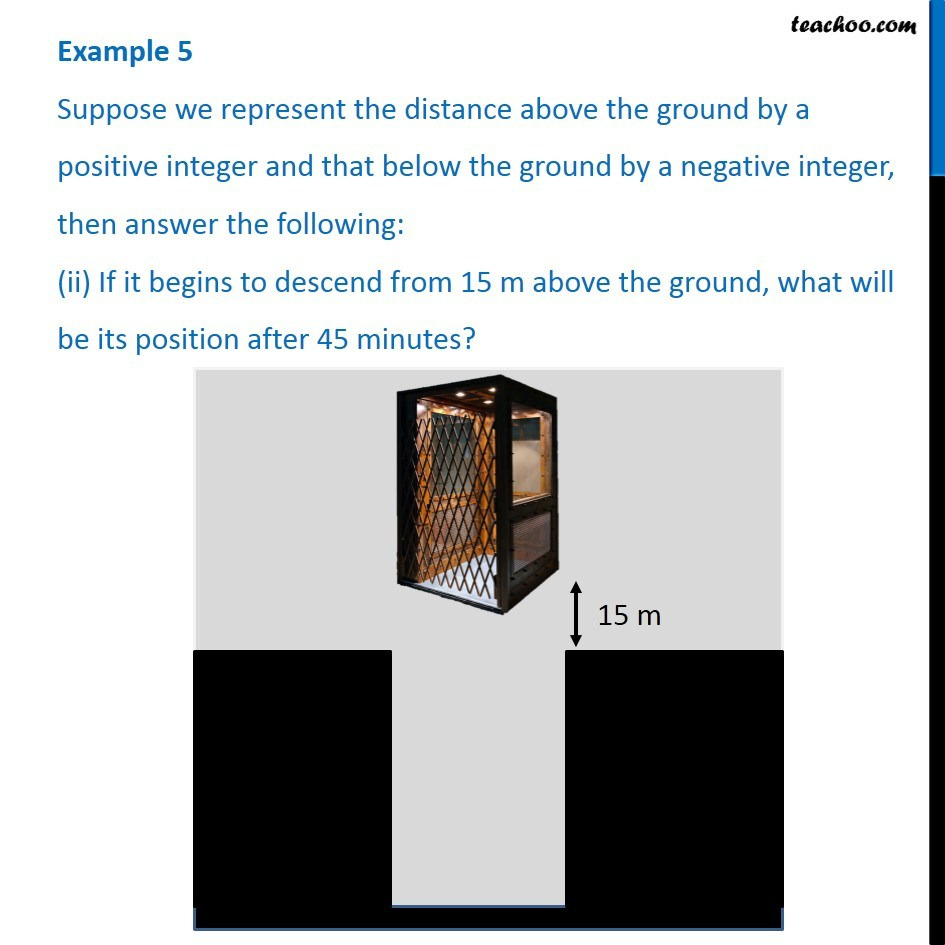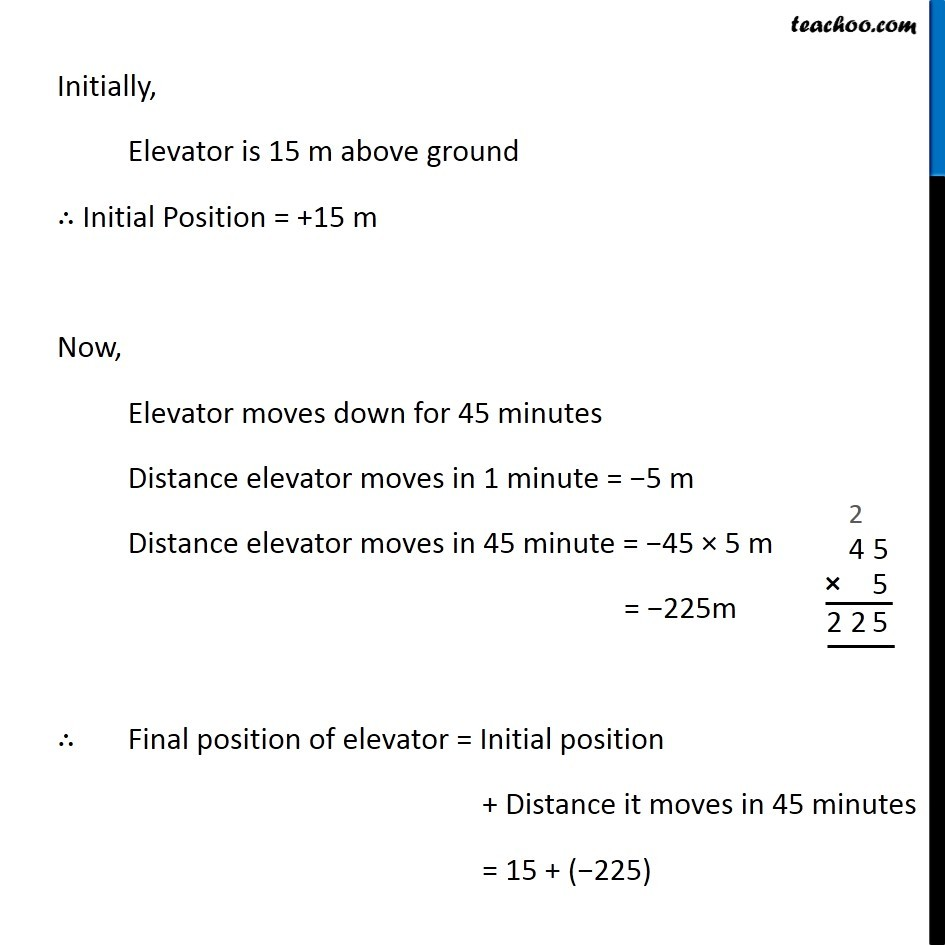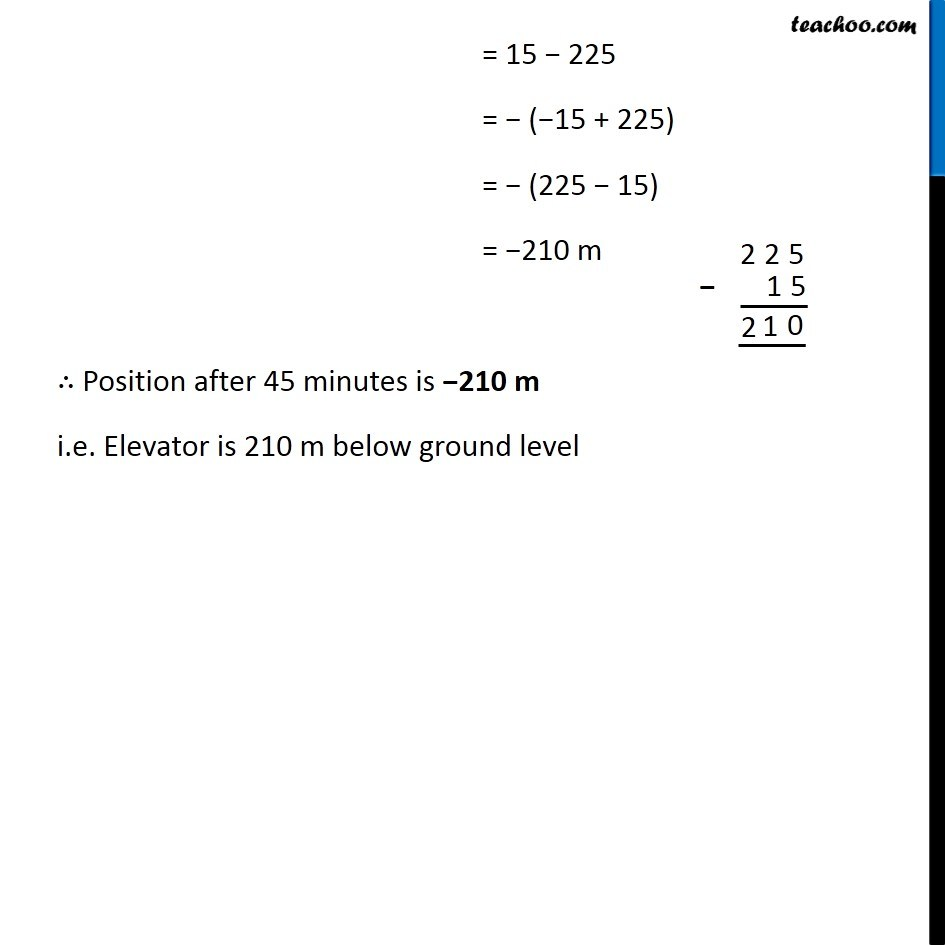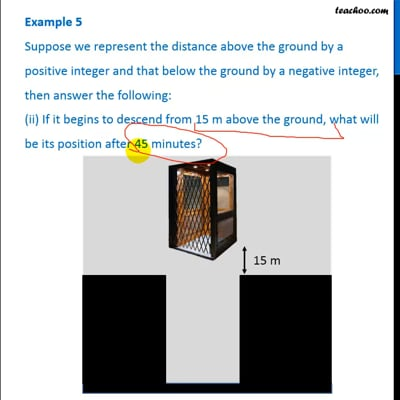This video is only available for Teachoo black users

### Transcript

Example 5 Suppose we represent the distance above the ground by a positive integer and that below the ground by a negative integer, then answer the following: (i) An elevator descends into a mine shaft at the rate of 5 metre per minute. What will be its position after one hour? Elevator goes down at rate of 5 metre per minute Therefore, Position of elevator after 1 minute = −5m Position of elevator after 60 minute = −5 × 60 m = −300 m Elevator is 300 m below ground level. Example 5 Suppose we represent the distance above the ground by a positive integer and that below the ground by a negative integer, then answer the following: (ii) If it begins to descend from 15 m above the ground, what will be its position after 45 minutes? Initially, Elevator is 15 m above ground ∴ Initial Position = +15 m Now, Elevator moves down for 45 minutes Distance elevator moves in 1 minute = −5 m Distance elevator moves in 45 minute = −45 × 5 m = −225m ∴ Final position of elevator = Initial position + Distance it moves in 45 minutes = 15 + (−225) = 15 − 225 = − (−15 + 225) = − (225 − 15) = −210 m ∴ Position after 45 minutes is −210 m i.e. Elevator is 210 m below ground level